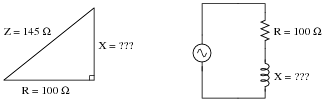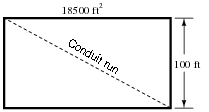# Trigonometry for AC Circuits

## Mathematics for Electronics

• #### Question 1Identify which trigonometric functions (sine, cosine, or tangent) are represented by each of the following ratios, with reference to the angle labeled with the Greek letter “Theta” (Θ):

 X R =

 X Z =

 R Z =

• #### Question 2Identify which trigonometric functions (sine, cosine, or tangent) are represented by each of the following ratios, with reference to the angle labeled with the Greek letter “Phi” (φ):

 R X =

 X Z =

 R Z =

• #### Question 3

The impedance triangle is often used to graphically relate Z, R, and X in a series circuit:Unfortunately, many students do not grasp the significance of this triangle, but rather memorize it as a “trick” used to calculate one of the three variables given the other two. Explain why a right triangle is an appropriate form to relate these variables, and what each side of the triangle actually represents.

• #### Question 4

Explain why the “impedance triangle” is not proper to use for relating total impedance, resistance, and reactance in parallel circuits as it is for series circuits:• #### Question 5

Examine the following circuits, then label the sides of their respective triangles with all the variables that are trigonometrically related in those circuits:• #### Question 6

Use the “impedance triangle” to calculate the necessary reactance of this series combination of resistance (R) and inductive reactance (X) to produce the desired total impedance of 145 Ω:Explain what equation(s) you use to calculate X, and the algebra necessary to achieve this result from a more common formula.

• #### Question 7

A series AC circuit exhibits a total impedance of 10 kΩ, with a phase shift of 65 degrees between voltage and current. Drawn in an impedance triangle, it looks like this:We know that the sine function relates the sides X and Z of this impedance triangle with the 65 degree angle, because the sine of an angle is the ratio of opposite to hypotenuse, with X being opposite the 65 degree angle. Therefore, we know we can set up the following equation relating these quantities together:

 sin65o = X Z

Solve this equation for the value of X, in ohms.

• #### Question 8

A series AC circuit exhibits a total impedance of 2.5 kΩ, with a phase shift of 30 degrees between voltage and current. Drawn in an impedance triangle, it looks like this:Use the appropriate trigonometric functions to calculate the equivalent values of R and X in this series circuit.

• #### Question 9

A parallel AC circuit draws 8 amps of current through a purely resistive branch and 14 amps of current through a purely inductive branch:Calculate the total current and the angle Θ of the total current, explaining your trigonometric method(s) of solution.

• #### Question 10

A parallel RC circuit has 10 μS of susceptance (B). How much conductance (G) is necessary to give the circuit a (total) phase angle of 22 degrees?• #### Question 11

The Pythagorean Theorem is used to calculate the length of the hypotenuse of a right triangle given the lengths of the other two sides:Write the standard form of the Pythagorean Theorem, and give an example of its use.

• #### Question 12

Use the “impedance triangle” to calculate the impedance of this series combination of resistance (R) and inductive reactance (X):Explain what equation(s) you use to calculate Z.

• #### Question 13

Trigonometric functions such as sine, cosine, and tangent are useful for determining the ratio of right-triangle side lengths given the value of an angle. However, they are not very useful for doing the reverse: calculating an angle given the lengths of two sides.Suppose we wished to know the value of angle Θ, and we happened to know the values of Z and R in this impedance triangle. We could write the following equation, but in its present form we could not solve for Θ:

 cosΘ = R Z

The only way we can algebraically isolate the angle Θ in this equation is if we have some way to “undo” the cosine function. Once we know what function will “undo” cosine, we can apply it to both sides of the equation and have Θ by itself on the left-hand side.

There is a class of trigonometric functions known as inverse or “arc” functions which will do just that: “undo” a regular trigonometric function so as to leave the angle by itself. Explain how we could apply an “arc-function” to the equation shown above to isolate Θ.

• #### Question 14

A series AC circuit contains 1125 ohms of resistance and 1500 ohms of reactance for a total circuit impedance of 1875 ohms. This may be represented graphically in the form of an impedance triangle:Since all side lengths on this triangle are known, there is no need to apply the Pythagorean Theorem. However, we may still calculate the two non-perpendicular angles in this triangle using “inverse” trigonometric functions, which are sometimes called arcfunctions.

Identify which arc-function should be used to calculate the angle Θ given the following pairs of sides:

 R and Z

 X and R

 X and Z

Show how three different trigonometric arcfunctions may be used to calculate the same angle Θ.

• #### Question 15

Students studying AC electrical theory become familiar with the impedance triangle very soon in their studies:What these students might not ordinarily discover is that this triangle is also useful for calculating electrical quantities other than impedance. The purpose of this question is to get you to discover some of the triangle’s other uses.

Fundamentally, this right triangle represents phasor addition, where two electrical quantities at right angles to each other (resistive versus reactive) are added together. In series AC circuits, it makes sense to use the impedance triangle to represent how resistance (R) and reactance (X) combine to form a total impedance (Z), since resistance and reactance are special forms of impedance themselves, and we know that impedances add in series.

List all of the electrical quantities you can think of that add (in series or in parallel) and then show how similar triangles may be drawn to relate those quantities together in AC circuits.

• #### Question 16

The Pythagorean Theorem is used to calculate the length of the hypotenuse of a right triangle given the lengths of the other two sides:Manipulate the standard form of the Pythagorean Theorem to produce a version that solves for the length of A given B and C, and also write a version of the equation that solves for the length of B given A and C.

• #### Question 17

A rectangular building foundation with an area of 18,500 square feet measures 100 feet along one side. You need to lay in a diagonal run of conduit from one corner of the foundation to the other. Calculate how much conduit you will need to make the run:Also, write an equation for calculating this conduit run length (L) given the rectangular area (A) and the length of one side (x).

• #### Question 18

Evaluate the length of side x in this right triangle, given the lengths of the other two sides:• #### Question 19

Evaluate the length of side x in this right triangle, given the lengths of the other two sides:• #### Question 20

Use a triangle to calculate the total voltage of the source for this series RC circuit, given the voltage drop across each component:Explain what equation(s) you use to calculate Vtotal, as well as why we must geometrically add these voltages together.

• #### Question 21

Use the “impedance triangle” to calculate the necessary resistance of this series combination of resistance (R) and inductive reactance (X) to produce the desired total impedance of 5.2 kΩ:Explain what equation(s) you use to calculate R, and the algebra necessary to achieve this result from a more common formula.

• #### Question 22

Use the “impedance triangle” to calculate the necessary reactance of this series combination of resistance (R) and capacitive reactance (X) to produce the desired total impedance of 300 Ω:Explain what equation(s) you use to calculate X, and the algebra necessary to achieve this result from a more common formula.

• #### Question 23

A parallel AC circuit draws 100 mA of current through a purely resistive branch and 85 mA of current through a purely capacitive branch:Calculate the total current and the angle Θ of the total current, explaining your trigonometric method(s) of solution.